# Unboxing JavaScript

We all have that model in our mind that a variable is a box containing some value e.g. let a contains 10.

Let me ask you a simple question. What is a variable?

You might have answered that variable is a box in memory that holds some value and you have pictured something as below in your mind.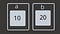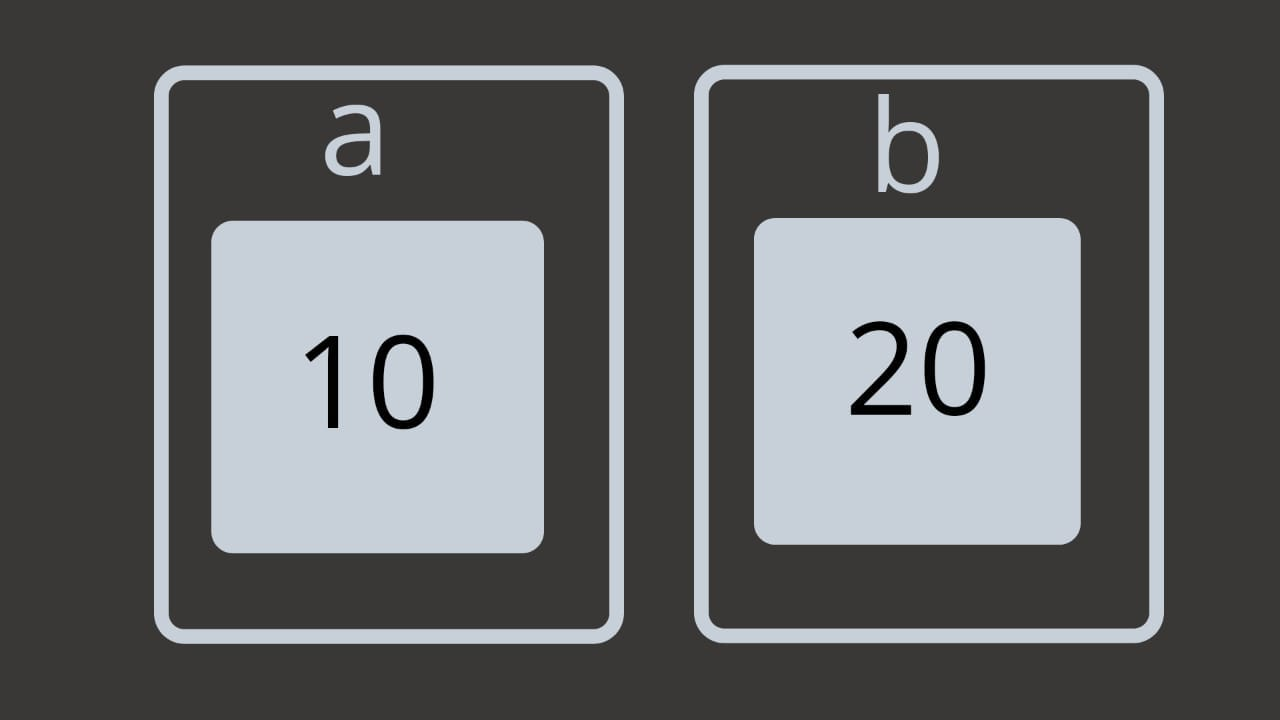Your general idea about variables.

But is that enough? Should we leave it just here?

No, to better understand the concepts of variables, objects, reference, etc in javascript we should dig a little deep to find out how this actually works.

Look at the illustration below and try to understand what is the difference in it with respect to one above.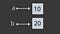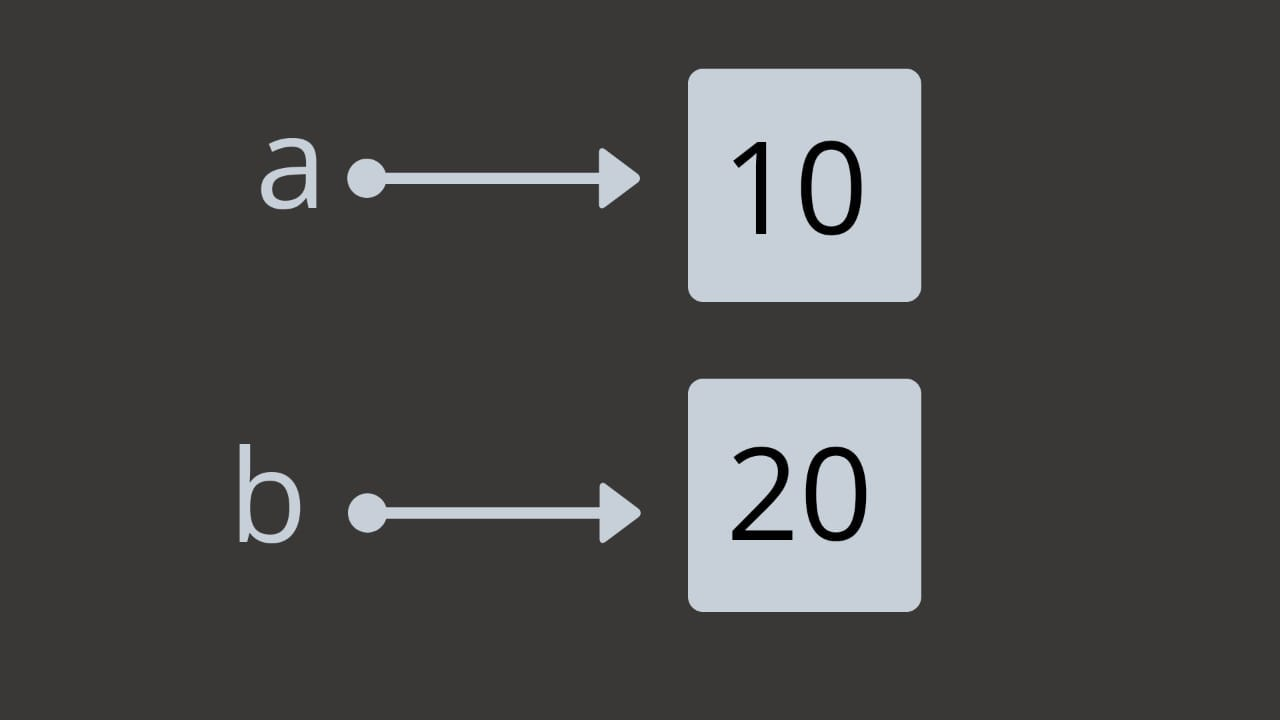We just unboxed the variable and the value.

A variable is just a reference to a value in memory. Imagine it like a thread from one point to another. So `a` is not `10` but it just points to `10` in the memory.

Consider the statements below :

`let a = 10;`

`a = 20;`

So does the value of `a` change? No, it is still the same thing just pointing to a new value. The illustration will help you to understand this better.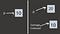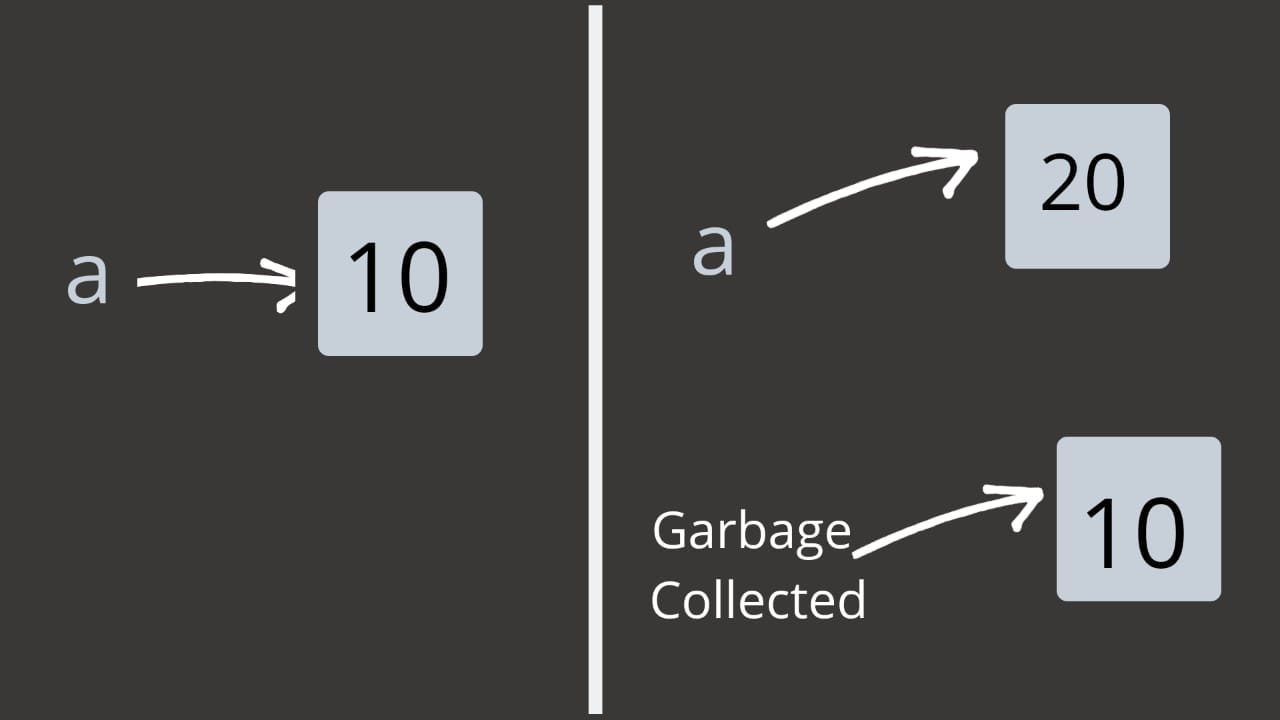This process is called autoboxing.

Now let’s move on the almighty objects in JavaScript.

Read the following example code: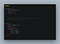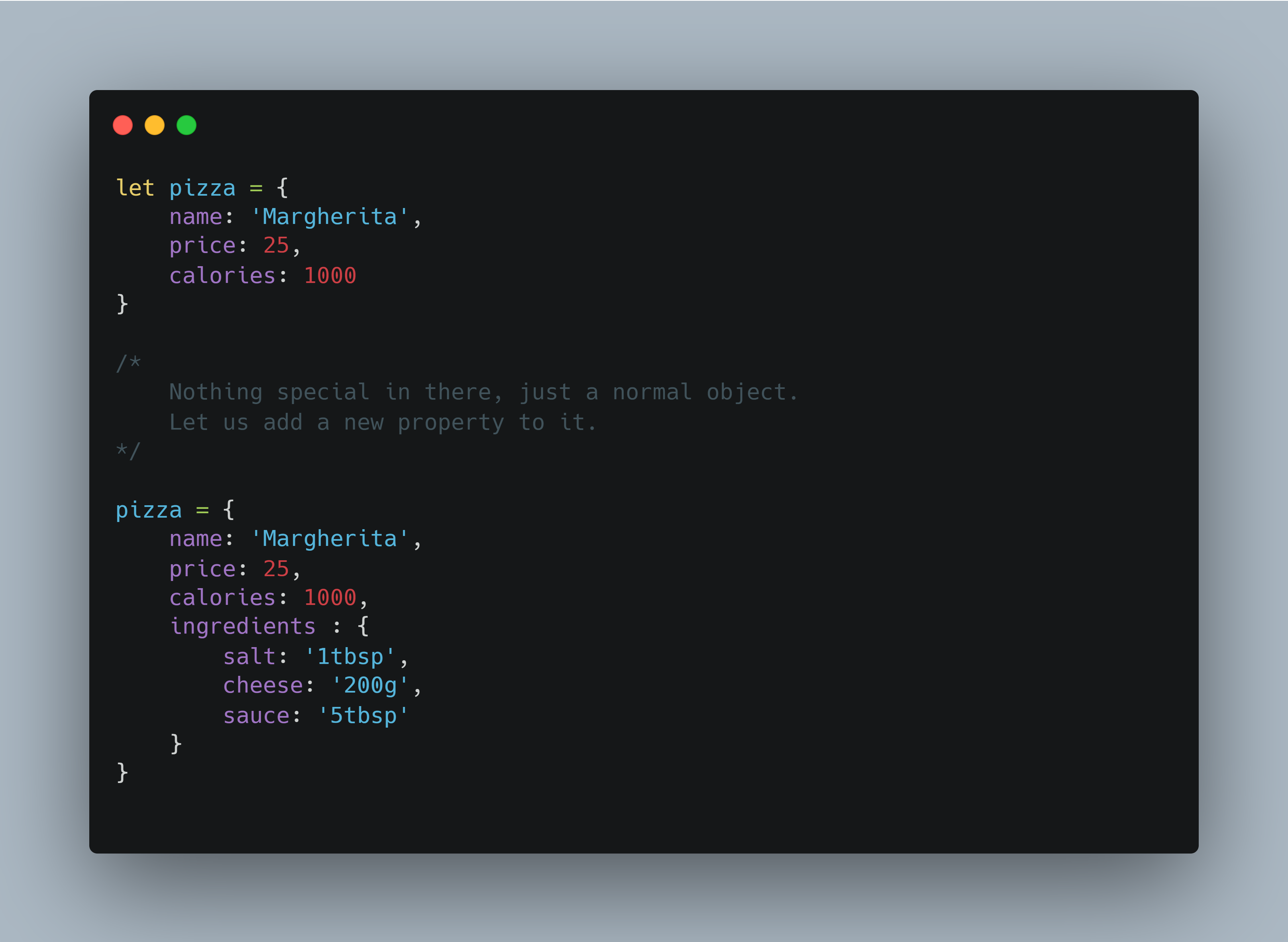Now try to imagine how this object will be stored in the memory. Hint: Try unboxing the pizza.

Done? So let’s see how this is actually represented.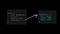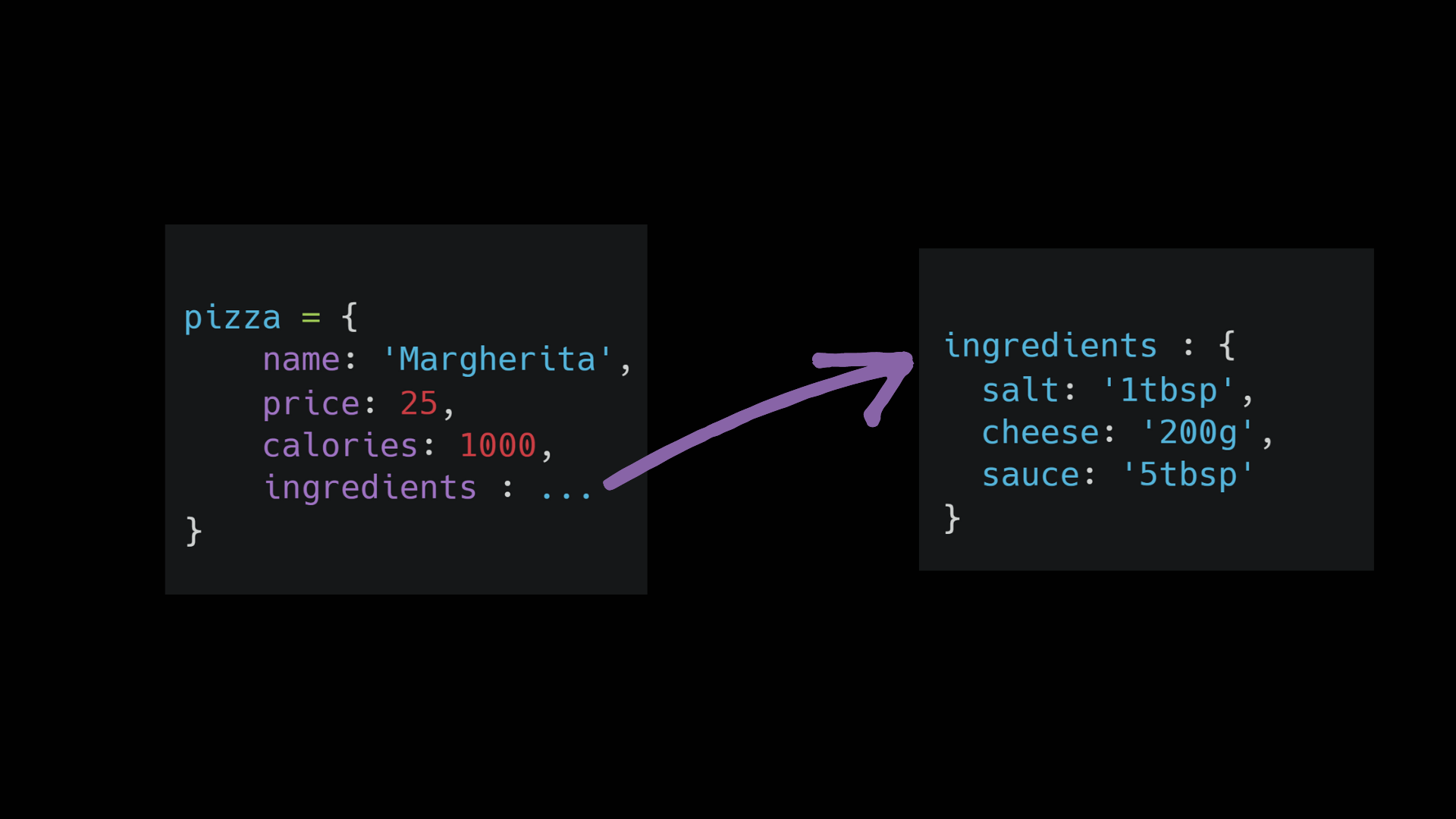Your model might look almost the same except that the ingredients object is nested inside the pizza object but this is not the case.

Objects cannot be nested in the memory so the ingredient object itself is a separate entity and is referenced by the pizza object.

But the question is why should you even care about this?

Try reading the code below and find the output of it.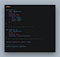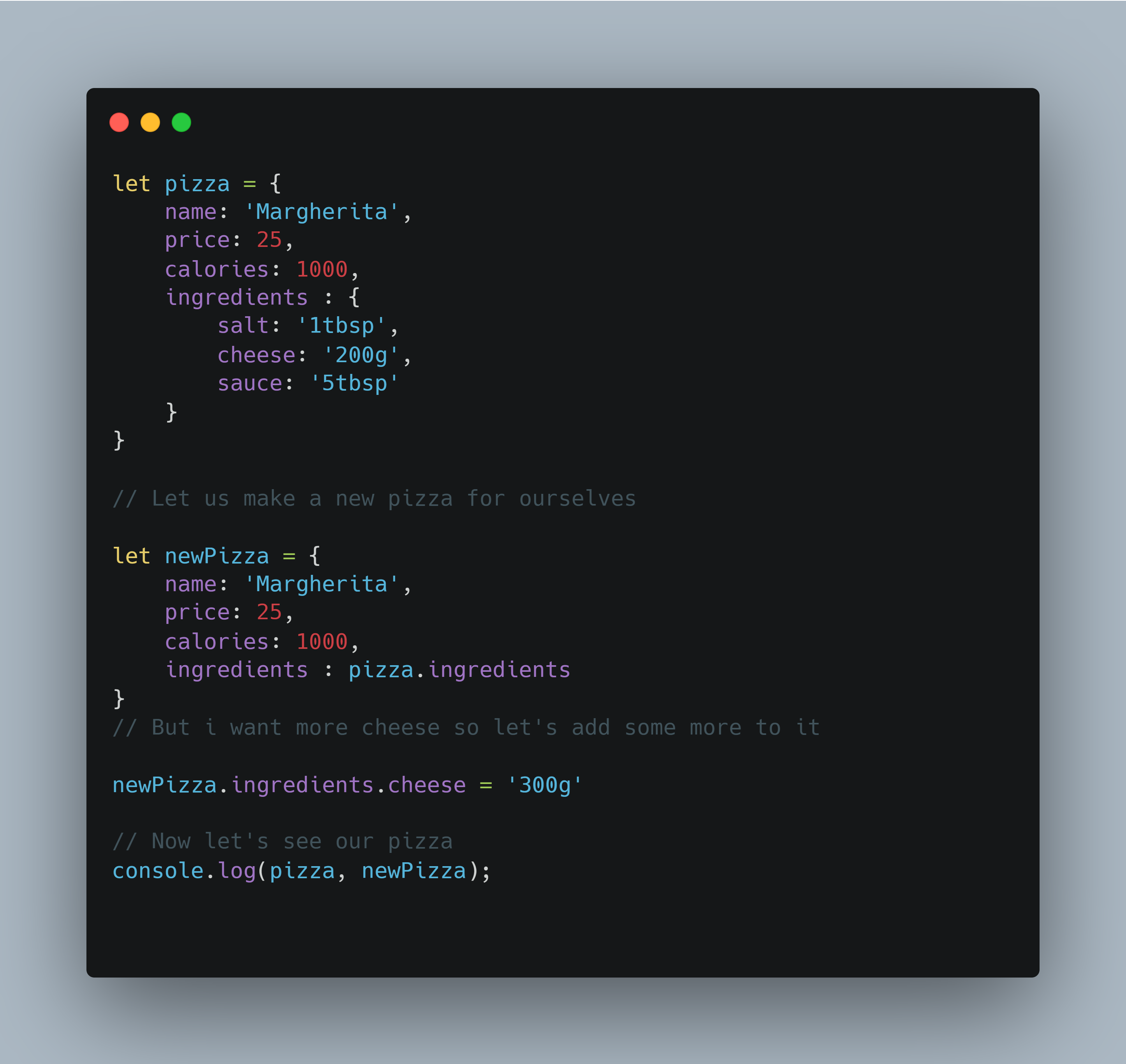What was your output?

Weird? Both of your pizzas are having 300g of cheese. To understand this just look at this illustration.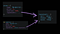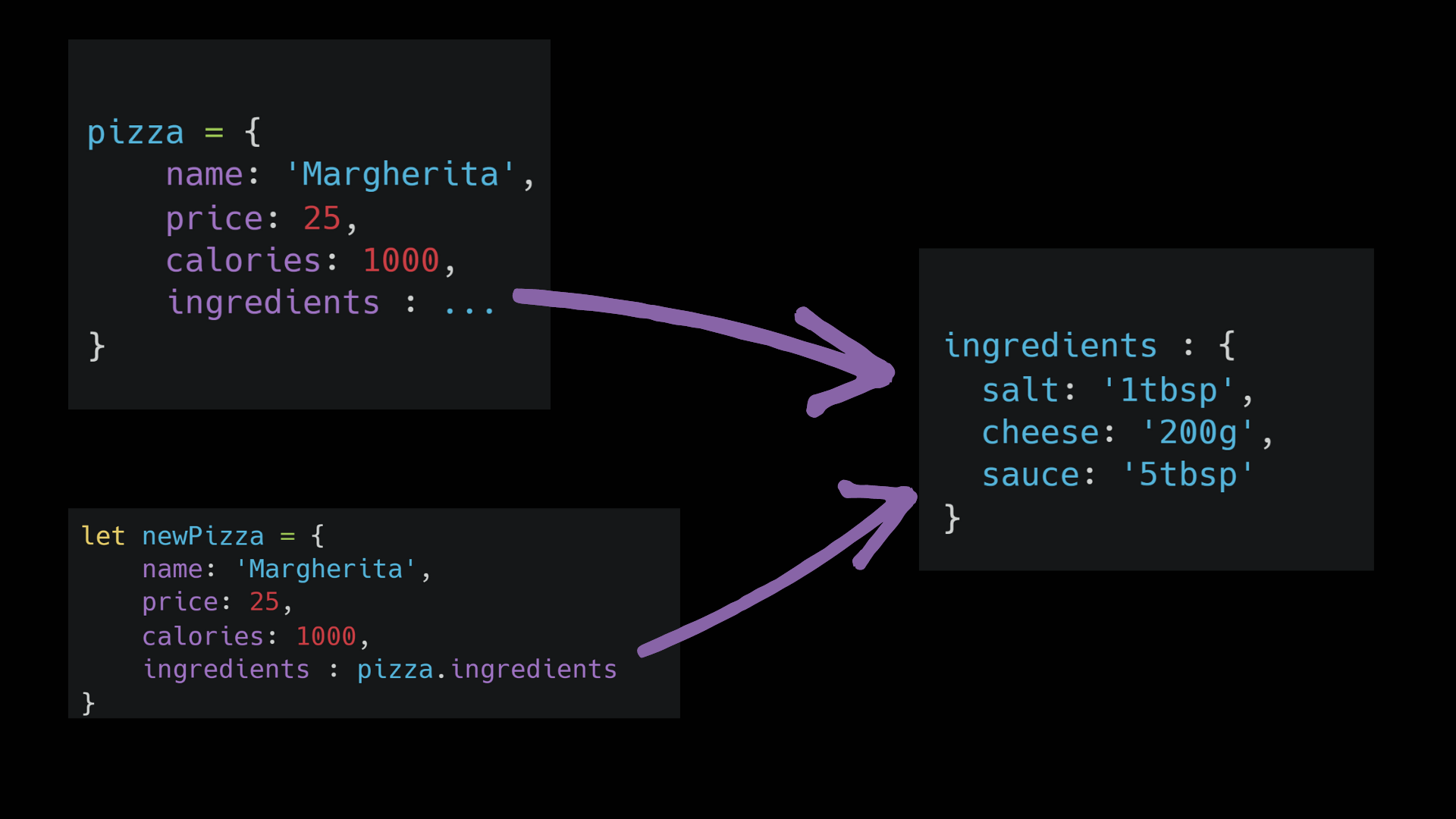Remember I told you that ingredients is a separate entity and is referenced by the `pizza` object. So when we created the `newPizza` we referenced the same entity and on changing the value in `newPizza` changed the entity itself. But both pizzas still referenced the same ingredients object.

So how to fix this? Actually, it’s quite simple.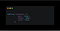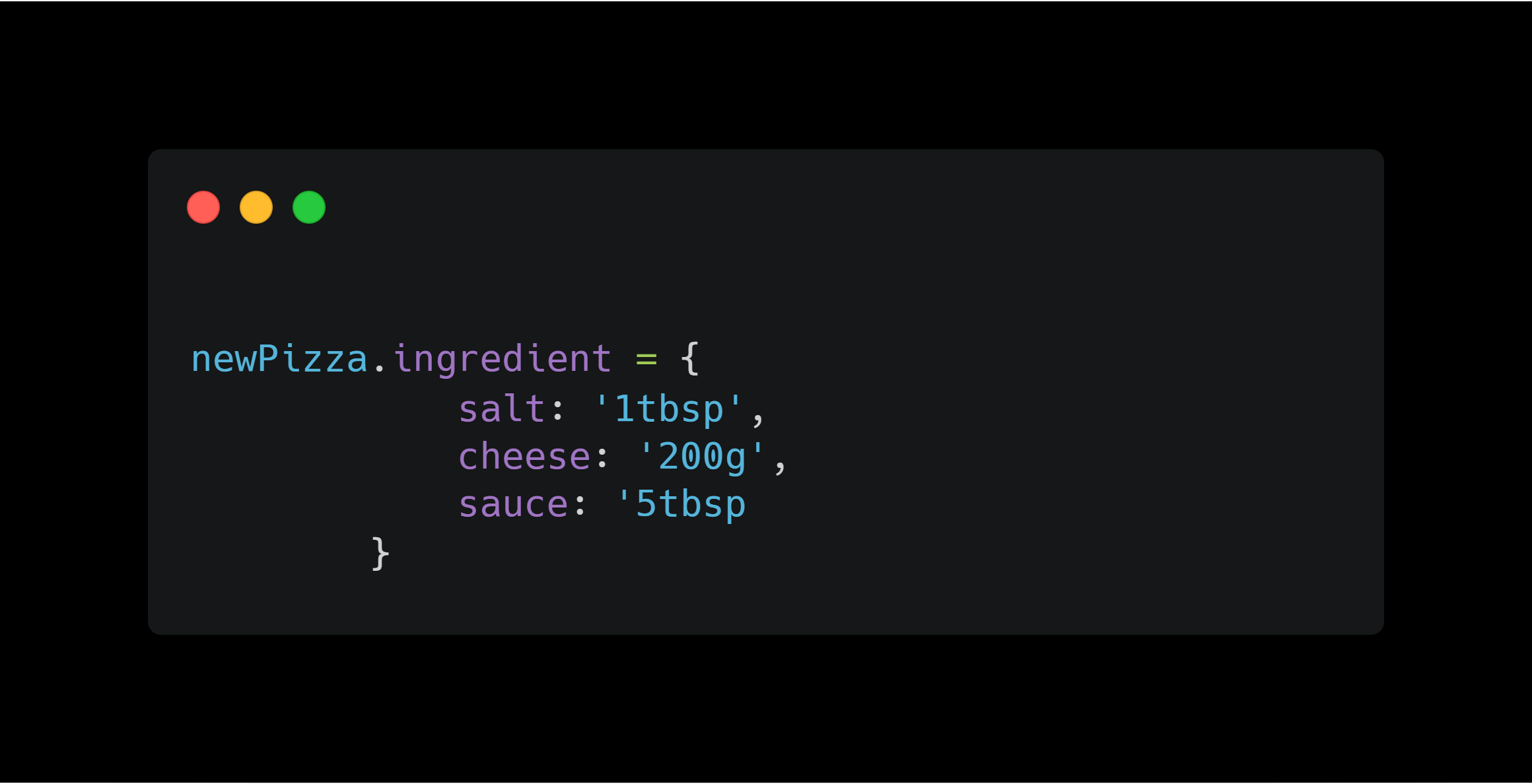Fixing the issue.

So rather than referencing the previous ingredients object, we created a new one that in itself is a separate entity. Have a look at the illustration below.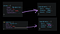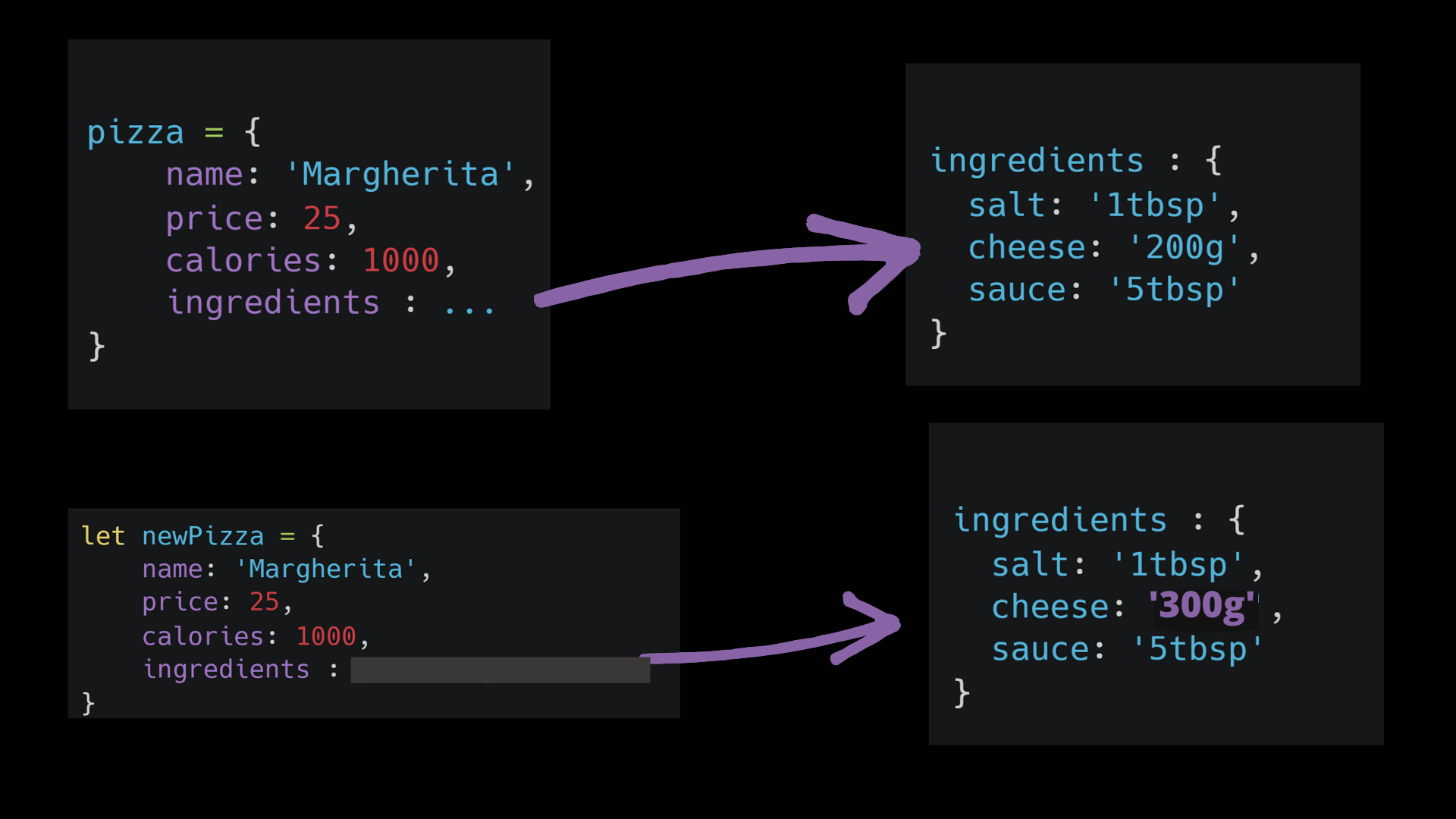Finally, we have unboxed the javascript.

“ I hope that article helped you to understand how basic thing generally works under the hood and in future, you could easily unbox the JavaScript easily.